Sample points on or in (sets of) spatial features. By default, returns a pre-specified number of points that is equal to size (if type = "random" and exact = TRUE) or an approximation of size otherwise. spatstat methods are interfaced and do not use the size argument, see examples.

## Usage

st_sample(x, size, ...)

# S3 method for sf
st_sample(x, size, ...)

# S3 method for sfc
st_sample(
x,
size,
...,
type = "random",
exact = TRUE,
warn_if_not_integer = TRUE,
by_polygon = FALSE,
progress = FALSE
)

# S3 method for sfg
st_sample(x, size, ...)

## Arguments

x

object of class sf or sfc

size

sample size(s) requested; either total size, or a numeric vector with sample sizes for each feature geometry. When sampling polygons, the returned sampling size may differ from the requested size, as the bounding box is sampled, and sampled points intersecting the polygon are returned.

...

passed on to sample for multipoint sampling, or to spatstat functions for spatstat sampling types (see details)

type

character; indicates the spatial sampling type; one of random, hexagonal (triangular really), regular, Fibonacci, or one of the spatstat methods such as Thomas for calling spatstat.random::rThomas (see Details).

exact

logical; should the length of output be exactly

warn_if_not_integer

logical; if FALSE then no warning is emitted if size is not an integer

by_polygon

logical; for MULTIPOLYGON geometries, should the effort be split by POLYGON? See https://github.com/r-spatial/sf/issues/1480 the same as specified by size? TRUE by default. Only applies to polygons, and when type = "random".

progress

logical; if TRUE show progress bar (only if size is a vector).

## Value

an sfc object containing the sampled POINT geometries

## Details

The function is vectorised: it samples size points across all geometries in the object if size is a single number, or the specified number of points in each feature if size is a vector of integers equal in length to the geometry of x.

if x has dimension 2 (polygons) and geographical coordinates (long/lat), uniform random sampling on the sphere is applied, see e.g. http://mathworld.wolfram.com/SpherePointPicking.html.

For regular or hexagonal sampling of polygons, the resulting size is only an approximation.

As parameter called offset can be passed to control ("fix") regular or hexagonal sampling: for polygons a length 2 numeric vector (by default: a random point from st_bbox(x)); for lines use a number like runif(1).

Fibonacci sampling see: Alvaro Gonzalez, 2010. Measurement of Areas on a Sphere Using Fibonacci and Latitude-Longitude Lattices. Mathematical Geosciences 42(1), p. 49-64

For regular sampling on the sphere, see also geosphere::regularCoordinates.

Sampling methods from package spatstat are interfaced (see examples), and need their own parameters to be set. For instance, to use spatstat.random::rThomas(), set type = "Thomas".

## Examples

nc = st_read(system.file("shape/nc.shp", package="sf"))
#> Reading layer nc' from data source
#>   /home/runner/work/_temp/Library/sf/shape/nc.shp' using driver ESRI Shapefile'
#> Simple feature collection with 100 features and 14 fields
#> Geometry type: MULTIPOLYGON
#> Dimension:     XY
#> Bounding box:  xmin: -84.32385 ymin: 33.88199 xmax: -75.45698 ymax: 36.58965
p1 = st_sample(nc[1:3, ], 6)
p2 = st_sample(nc[1:3, ], 1:3)
plot(st_geometry(nc)[1:3])
plot(p2, add = TRUE, pch = 2)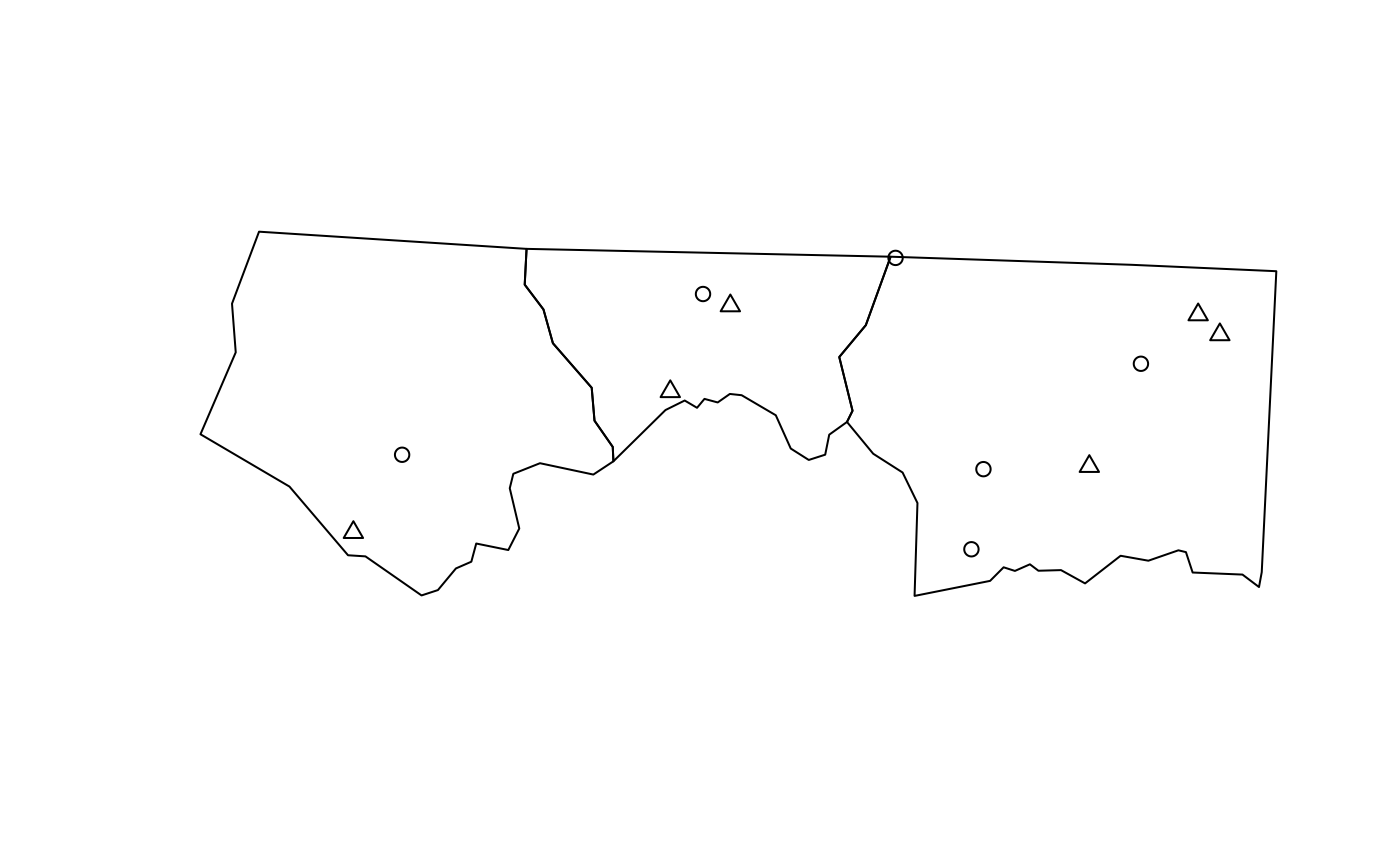x = st_sfc(st_polygon(list(rbind(c(0,0),c(90,0),c(90,90),c(0,90),c(0,0)))), crs = st_crs(4326))
plot(x, axes = TRUE, graticule = TRUE)
if (sf_extSoftVersion()["proj.4"] >= "4.9.0")
plot(p <- st_sample(x, 1000), add = TRUE)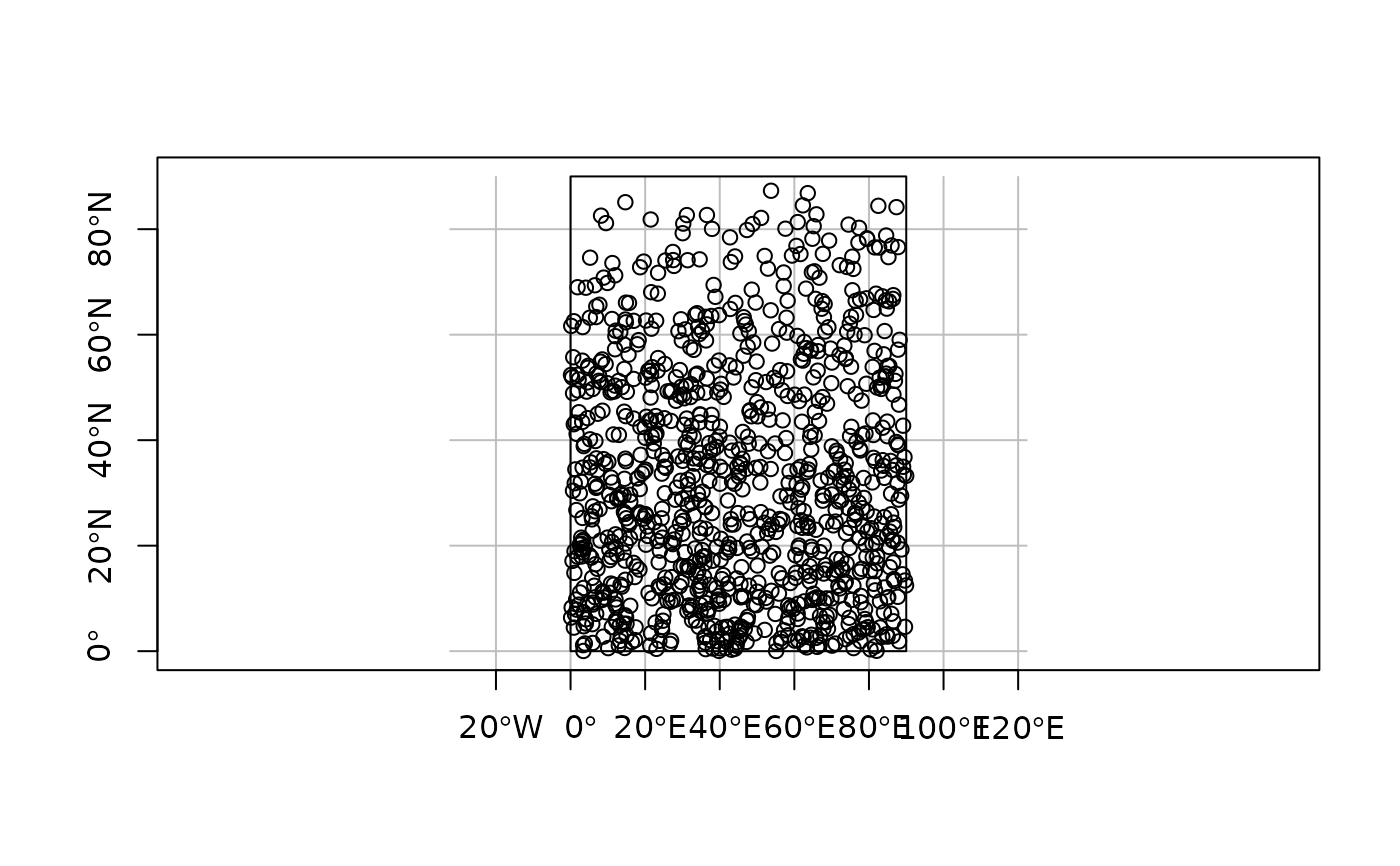if (require(lwgeom, quietly = TRUE)) { # for st_segmentize()
x2 = st_transform(st_segmentize(x, 1e4), st_crs("+proj=ortho +lat_0=30 +lon_0=45"))
g = st_transform(st_graticule(), st_crs("+proj=ortho +lat_0=30 +lon_0=45"))
plot(x2, graticule = g)
if (sf_extSoftVersion()["proj.4"] >= "4.9.0") {
p2 = st_transform(p, st_crs("+proj=ortho +lat_0=30 +lon_0=45"))
}
}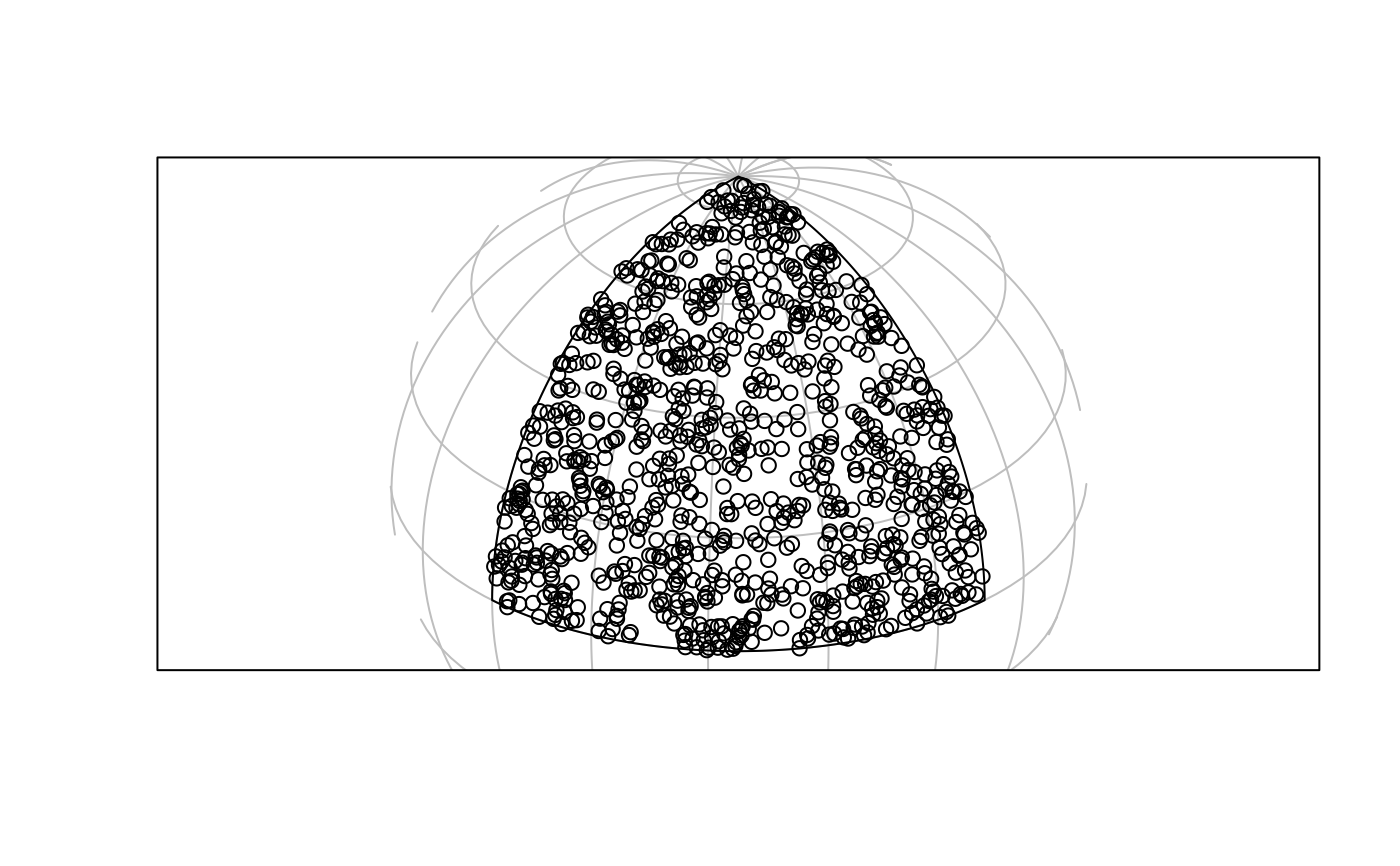x = st_sfc(st_polygon(list(rbind(c(0,0),c(90,0),c(90,10),c(0,90),c(0,0))))) # NOT long/lat:
plot(x)
p_exact = st_sample(x, 1000, exact = TRUE)
p_not_exact = st_sample(x, 1000, exact = FALSE)
length(p_exact); length(p_not_exact)
#>  1000
#>  1000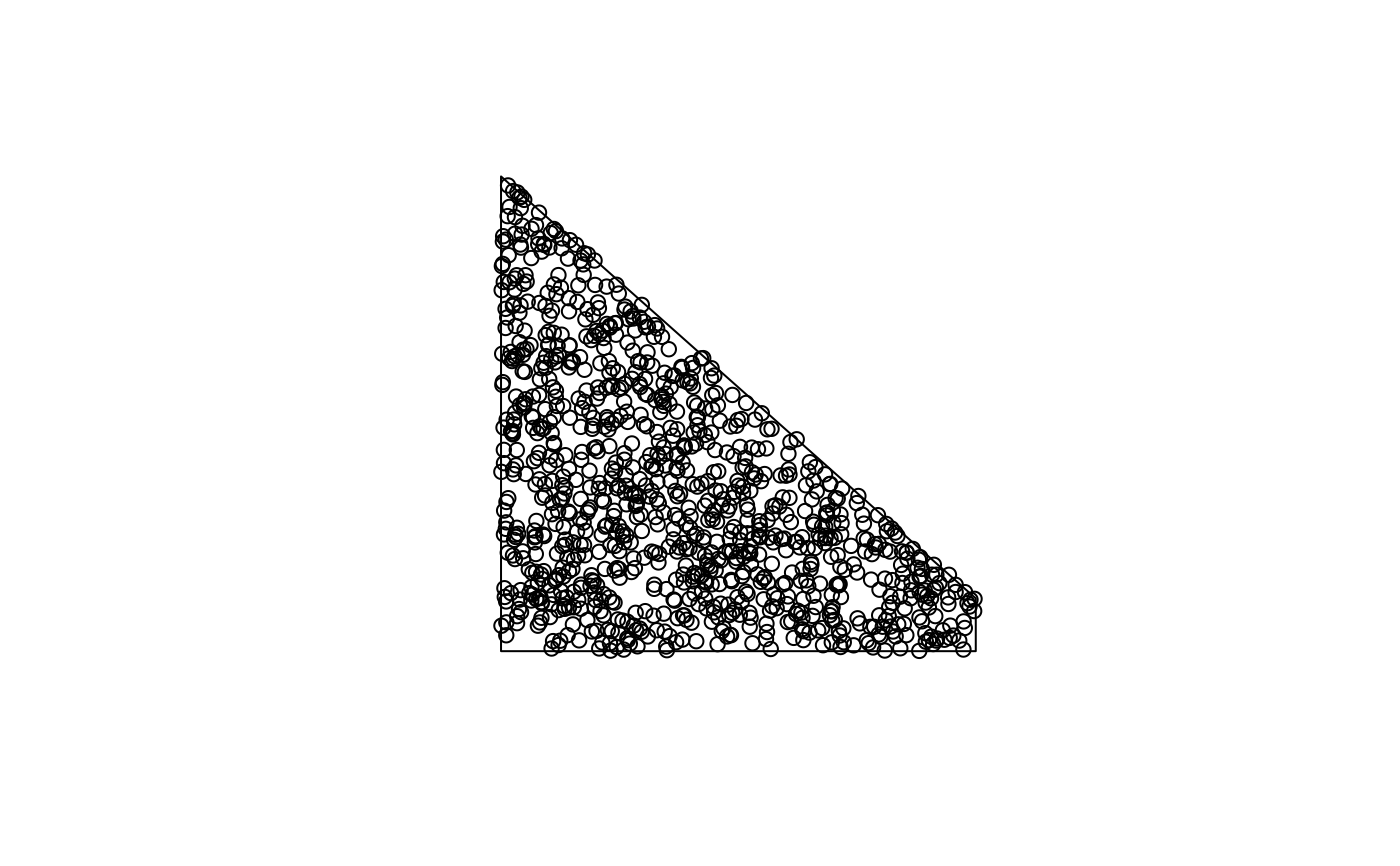x = st_sfc(st_polygon(list(rbind(c(-180,-90),c(180,-90),c(180,90),c(-180,90),c(-180,-90)))),
crs=st_crs(4326))
# FIXME:
#if (sf_extSoftVersion()["proj.4"] >= "4.9.0") {
#  p = st_sample(x, 1000)
#  st_sample(p, 3)
#}
# hexagonal:
sfc = st_sfc(st_polygon(list(rbind(c(0,0), c(1,0), c(1,1), c(0,0)))))
plot(sfc)
h = st_sample(sfc, 100, type = "hexagonal")
h1 = st_sample(sfc, 100, type = "hexagonal")
plot(h1, col = 'red', add = TRUE)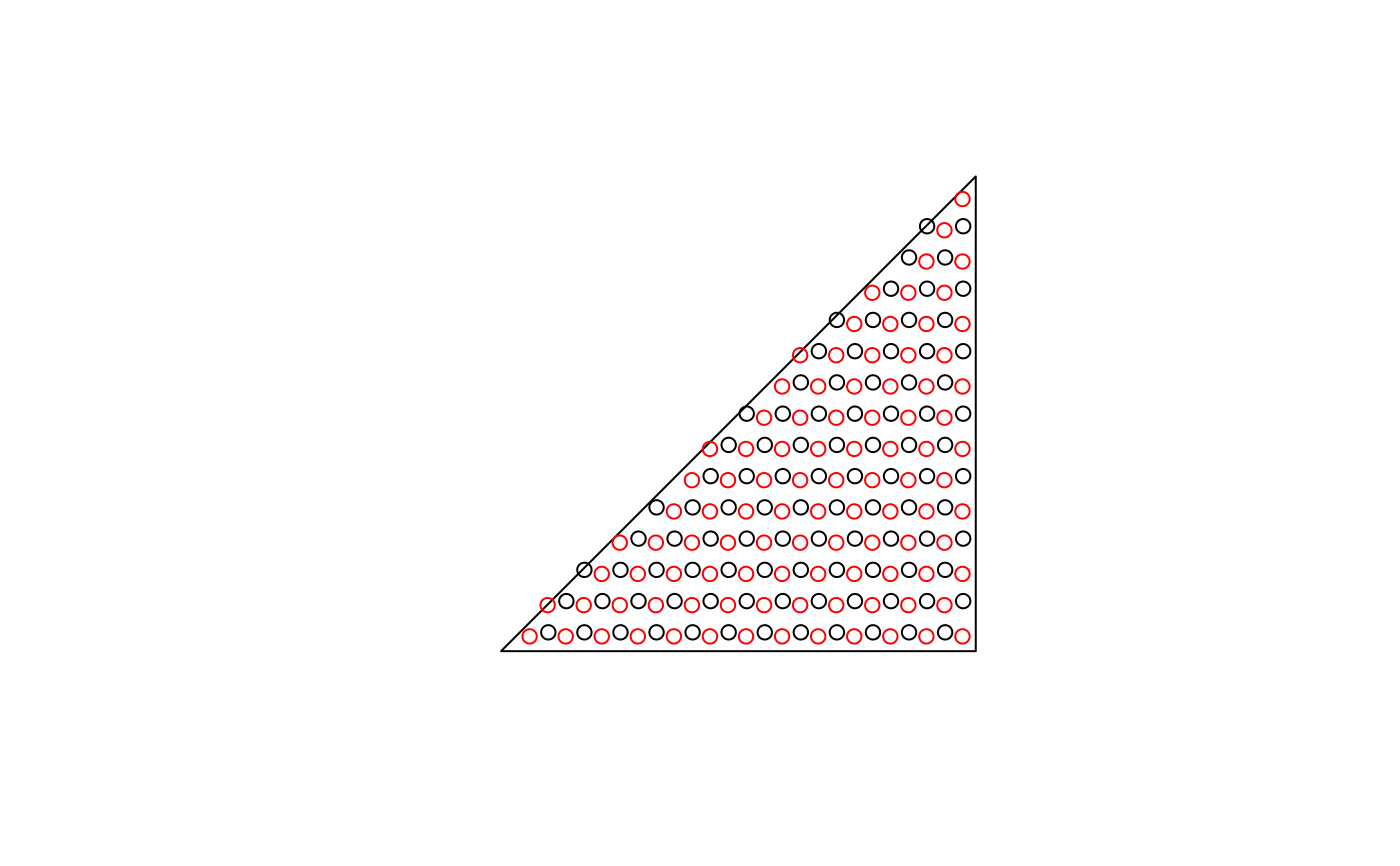c(length(h), length(h1)) # approximate!
#>  97 99
pt = st_multipoint(matrix(1:20,,2))
ls = st_sfc(st_linestring(rbind(c(0,0),c(0,1))),
st_linestring(rbind(c(0,0),c(.1,0))),
st_linestring(rbind(c(0,1),c(.1,1))),
st_linestring(rbind(c(2,2),c(2,2.00001))))
st_sample(ls, 80)
#> Geometry set for 4 features  (with 1 geometry empty)
#> Geometry type: MULTIPOINT
#> Dimension:     XY
#> Bounding box:  xmin: 0 ymin: 0 xmax: 0.08531849 ymax: 1
#> CRS:           NA
#> MULTIPOINT ((0 0.01737041), (0 0.8441574), (0 0...
#> MULTIPOINT ((0.03337024 0), (0.03010541 0), (0....
#> MULTIPOINT ((0.04159846 1), (0.06942899 1), (0....
#> MULTIPOINT EMPTY
plot(st_sample(ls, 80))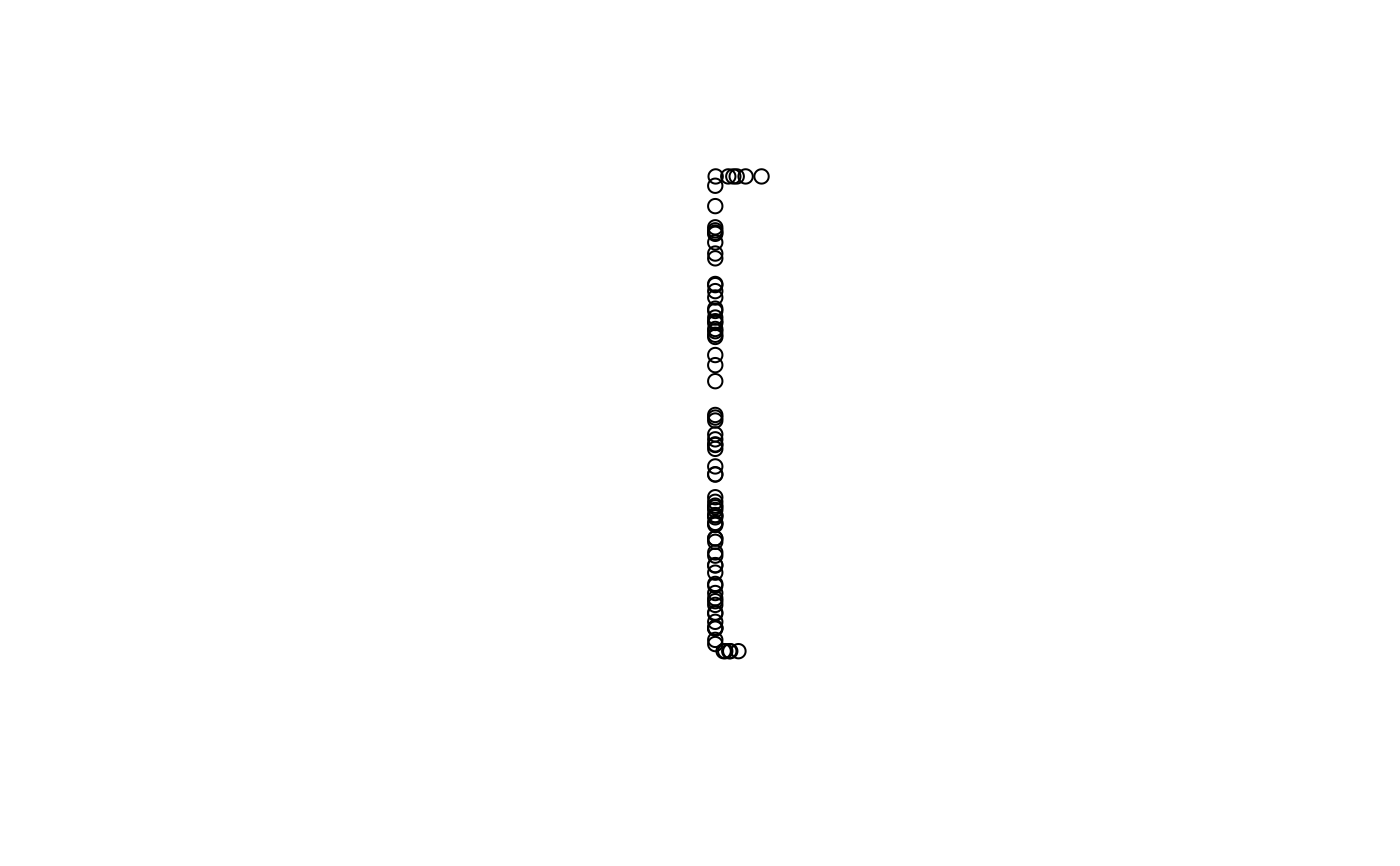# spatstat example:
if (require(spatstat.random)) {
x <- sf::st_sfc(sf::st_polygon(list(rbind(c(0, 0), c(10, 0), c(10, 10), c(0, 0)))))
# for spatstat.random::rThomas(), set type = "Thomas":
pts <- st_sample(x, kappa = 1, mu = 10, scale = 0.1, type = "Thomas")
}
`